# Surface tension facts for kids

Kids Encyclopedia Facts
Mechanics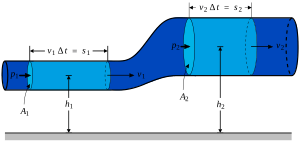Fluid mechanics
Fluids
Fluid statics · Fluid dynamics
Surface tension
Navier–Stokes equations
Viscosity:
Newtonian, Non-Newtonian

Surface tension is an effect where the surface of a liquid is strong. The surface can hold up a weight, and the surface of a water droplet holds the droplet together, in a ball shape. Some small things can float on a surface because of surface tension, even though they normally could not float. Some insects (e.g. water striders) can run on the surface of water because of this. This property is caused by the molecules in the liquid being attracted to each other (cohesion), and is responsible for many of the behaviors of liquids.

Surface tension has the dimension of force per unit length, or of energy per unit area. The two are equivalent—but when referring to energy per unit of area, people use the term surface energy—which is a more general term in the sense that it applies also to solids and not just liquids.

In materials science, surface tension is used for either surface stress or surface free energy.

## Causes

The cohesive forces among the liquid molecules cause surface tension. In the bulk of the liquid, each molecule is pulled equally in every direction by neighboring liquid molecules, resulting in a net force of zero. The molecules at the surface do not have other molecules on all sides of them and therefore are pulled inwards. This creates some internal pressure and forces liquid surfaces to contract to the minimal area.

Surface tension is responsible for the shape of liquid droplets. Although easily deformed, droplets of water tend to be pulled into a spherical shape by the cohesive forces of the surface layer. In the absence of other forces, including gravity, drops of virtually all liquids would be perfectly spherical. The spherical shape minimizes the necessary "wall tension" of the surface layer according to Laplace's law.

Another way to view it is in terms of energy. A molecule in contact with a neighbor is in a lower state of energy than if it were alone (not in contact with a neighbor). The interior molecules have as many neighbors as they can possibly have, but the boundary molecules are missing neighbors (compared to interior molecules). So, the boundary molecules have a higher energy. For the liquid to minimize its energy state, the number of higher energy boundary molecules must be minimized. The minimized quantity of boundary molecules results in a minimized surface area.

As a result of surface area minimization, a surface will assume the smoothest shape it can. Any curvature in the surface shape results in greater area and a higher energy. So, the surface will push back against any curvature in much the same way as a ball pushed uphill will push back to minimize its gravitational potential energy.

## Effects in everyday life

### Water

Studying water shows several effects of surface tension:

A. Rain water forms beads on the surface of a waxy surface, such as a leaf. Water adheres weakly to wax and strongly to itself, so water clusters into drops. Surface tension gives them their near-spherical shape, because a sphere has the smallest possible surface area to volume ratio.

B. Formation of drops occurs when a mass of liquid is stretched. The animation shows water adhering to the faucet gaining mass until it is stretched to a point where the surface tension can no longer bind it to the faucet. It then separates and surface tension forms the drop into a sphere. If a stream of water were running from the faucet, the stream would break up into drops during its fall. Gravity stretches the stream, then surface tension pinches it into spheres.

C. Objects denser than water still float when the object is nonwettable and its weight is small enough to be borne by the forces arising from surface tension. For example, water striders use surface tension to walk on the surface of a pond. The surface of the water behaves like an elastic film: the insect's feet cause indentations in the water's surface, increasing its surface area.

D. Separation of oil and water (in this case, water and liquid wax) is caused by a tension in the surface between dissimilar liquids. This type of surface tension is called "interface tension", but its physics are the same.

E. Tears of wine is the formation of drops and rivulets on the side of a glass containing an alcoholic beverage. Its cause is a complex interaction between the differing surface tensions of water and ethanol. It is induced by a combination of surface tension modification of water by ethanol together with ethanol evaporating faster than water.

### Surfactants

Surface tension is visible in other common phenomena, especially when surfactants are used to decrease it:

• Soap bubbles have very large surface areas with very little mass. Bubbles in pure water are unstable. The addition of surfactants, however, can have a stabilizing effect on the bubbles (see Marangoni effect). Notice that surfactants actually reduce the surface tension of water by a factor of three or more.
• Emulsions are a type of solution in which surface tension plays a role. Tiny fragments of oil suspended in pure water will spontaneously assemble themselves into much larger masses. But the presence of a surfactant provides a decrease in surface tension, which permits stability of minute droplets of oil in the bulk of water (or vice versa).

## Basic physics

### Two definitions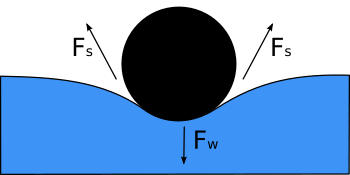Diagram shows, in cross-section, a needle floating on the surface of water. Its weight, Fw, depresses the surface, and is balanced by the surface tension forces on either side, Fs, which are each parallel to the water's surface at the points where it contacts the needle. Notice that the horizontal components of the two Fs arrows point in opposite directions, so they cancel each other, but the vertical components point in the same direction and therefore add up to balance Fw.

Surface tension, represented by the symbol γ is defined as the force along a line of unit length, where the force is parallel to the surface but perpendicular to the line. One way to picture this is to imagine a flat soap film bounded on one side by a taut thread of length, L. The thread will be pulled toward the interior of the film by a force equal to 2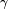$\scriptstyle\gamma$L (the factor of 2 is because the soap film has two sides, hence two surfaces). Surface tension is therefore measured in forces per unit length. Its SI unit is newton per meter but the cgs unit of dyne per cm is also used. One dyn/cm corresponds to 0.001 N/m.

An equivalent definition, one that is useful in thermodynamics, is work done per unit area. As such, in order to increase the surface area of a mass of liquid by an amount, δA, a quantity of work,$\scriptstyle\gamma$δA, is needed. This work is stored as potential energy. Consequently surface tension can be also measured in SI system as joules per square meter and in the cgs system as ergs per cm2. Since mechanical systems try to find a state of minimum potential energy, a free droplet of liquid naturally assumes a spherical shape, which has the minimum surface area for a given volume.

The equivalence of measurement of energy per unit area to force per unit length can be proven by dimensional analysis.

### Surface curvature and pressure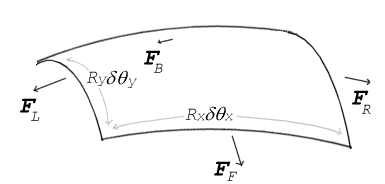Surface tension forces acting on a tiny (differential) patch of surface. δθx and δθy indicate the amount of bend over the dimensions of the patch. Balancing the tension forces with pressure leads to the Young–Laplace equation

If no force acts normal to a tensioned surface, the surface must remain flat. But if the pressure on one side of the surface differs from pressure on the other side, the pressure difference times surface area results in a normal force. In order for the surface tension forces to cancel the force due to pressure, the surface must be curved. The diagram shows how surface curvature of a tiny patch of surface leads to a net component of surface tension forces acting normal to the center of the patch. When all the forces are balanced, the resulting equation is known as the Young–Laplace equation: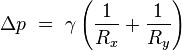$\Delta p\ =\ \gamma \left( \frac{1}{R_x} + \frac{1}{R_y} \right)$

where:

• Δp is the pressure difference.
•$\scriptstyle\gamma$ is surface tension.
• Rx and Ry are radii of curvature in each of the axes that are parallel to the surface.

The quantity in parentheses on the right hand side is in fact (twice) the mean curvature of the surface (depending on normalization).

Solutions to this equation determine the shape of water drops, puddles, menisci, soap bubbles, and all other shapes determined by surface tension. (Another example is the shape of the impressions that a water strider's feet make on the surface of a pond).

The table below shows how the internal pressure of a water droplet increases with decreasing radius. For not very small drops the effect is subtle, but the pressure difference becomes enormous when the drop sizes approach the molecular size. (In the limit of a single molecule the concept becomes meaningless.)

Δp for water drops of different radii at STP
Droplet radius 1 mm 0.1 mm 1 μm 10 nm
Δp (atm) 0.0014 0.0144 1.436 143.6

### Liquid surface

It is hard to find the shape of the minimal surface bounded by some arbitrary shaped frame using just mathematics. Yet by fashioning the frame out of wire and dipping it in soap-solution, a locally minimal surface will appear in the resulting soap-film within seconds.

The reason for this is that the pressure difference across a fluid interface is proportional to the mean curvature, as seen in the Young-Laplace equation. For an open soap film, the pressure difference is zero, hence the mean curvature is zero, and minimal surfaces have the property of zero mean curvature.

### Contact angles

The surface of any liquid is an interface between that liquid and some other medium. The top surface of a pond, for example, is an interface between the pond water and the air. Surface tension, then, is not a property of the liquid alone, but a property of the liquid's interface with another medium. If a liquid is in a container, then besides the liquid/air interface at its top surface, there is also an interface between the liquid and the walls of the container. The surface tension between the liquid and air is usually different (greater than) its surface tension with the walls of a container. Where the two surfaces meet, the geometry will balance all forces.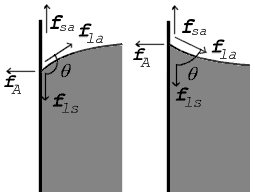Forces at contact point shown for contact angle greater than 90° (left) and less than 90° (right)

Where the two surfaces meet, they form a contact angle,$\theta$, which is the angle the tangent to the surface makes with the solid surface. The diagram to the right shows two examples. Tension forces are shown for the liquid-air interface, the liquid-solid interface, and the solid-air interface. The example on the left is where the difference between the liquid-solid and solid-air surface tension,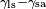$\gamma_{\mathrm{ls}} - \gamma_{\mathrm{sa}}$, is less than the liquid-air surface tension,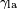$\gamma_{\mathrm{la}}$, but is still positive, that is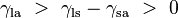$\gamma_{\mathrm{la}}\ >\ \gamma_{\mathrm{ls}} - \gamma_{\mathrm{sa}}\ >\ 0$

In the diagram, both the vertical and horizontal forces must cancel exactly at the contact point, known as equilibrium. The horizontal component of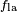$f_\mathrm{la}$ is canceled by the adhesive force,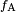$f_\mathrm{A}$.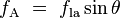$f_\mathrm{A}\ =\ f_\mathrm{la} \sin \theta$

The more important balance of forces, though, is in the vertical direction. The vertical component of$f_\mathrm{la}$ must exactly cancel the force,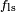$f_\mathrm{ls}$.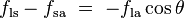$f_\mathrm{ls} - f_\mathrm{sa}\ =\ -f_\mathrm{la} \cos \theta$
Liquid Solid Contact angle
water
 soda-lime glass lead glass fused quartz
ethanol
diethyl ether
carbon tetrachloride
glycerol
acetic acid
water paraffin wax 107°
silver 90°
methyl iodide soda-lime glass 29°
fused quartz 33°
mercury soda-lime glass 140°
Some liquid-solid contact angles

Since the forces are in direct proportion to their respective surface tensions, we also have: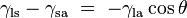$\gamma_\mathrm{ls} - \gamma_\mathrm{sa}\ =\ -\gamma_\mathrm{la} \cos \theta$

where

•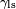$\gamma_\mathrm{ls}$ is the liquid-solid surface tension,
•$\gamma_\mathrm{la}$ is the liquid-air surface tension,
•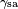$\gamma_\mathrm{sa}$ is the solid-air surface tension,
•$\theta$ is the contact angle, where a concave meniscus has contact angle less than 90° and a convex meniscus has contact angle of greater than 90°.

This means that although the difference between the liquid-solid and solid-air surface tension,$\gamma_\mathrm{ls} - \gamma_\mathrm{sa}$, is difficult to measure directly, it can be inferred from the liquid-air surface tension,$\gamma_\mathrm{la}$, and the equilibrium contact angle,$\theta$, which is a function of the easily measurable advancing and receding contact angles (see main article contact angle).

This same relationship exists in the diagram on the right. But in this case we see that because the contact angle is less than 90°, the liquid-solid/solid-air surface tension difference must be negative: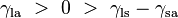$\gamma_\mathrm{la}\ >\ 0\ >\ \gamma_\mathrm{ls} - \gamma_\mathrm{sa}$

#### Special contact angles

Observe that in the special case of a water-silver interface where the contact angle is equal to 90°, the liquid-solid/solid-air surface tension difference is exactly zero.

Another special case is where the contact angle is exactly 180°. Water with specially prepared Teflon approaches this. Contact angle of 180° occurs when the liquid-solid surface tension is exactly equal to the liquid-air surface tension.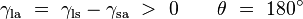$\gamma_{\mathrm{la}}\ =\ \gamma_{\mathrm{ls}} - \gamma_\mathrm{sa}\ >\ 0\qquad \theta\ =\ 180^\circ$

## Methods of measurement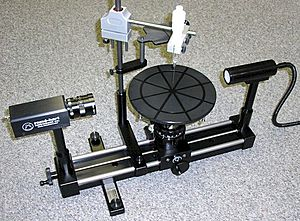Surface tension can be measured using the pendant drop method on a goniometer.

Because surface tension manifests itself in various effects, it offers a number of paths to its measurement. Which method is optimal depends upon the nature of the liquid being measured, the conditions under which its tension is to be measured, and the stability of its surface when it is deformed.

• Du Noüy Ring method: The traditional method used to measure surface or interfacial tension. Wetting properties of the surface or interface have little influence on this measuring technique. Maximum pull exerted on the ring by the surface is measured.
• Du Noüy-Padday method: A minimized version of Du Noüy method uses a small diameter metal needle instead of a ring, in combination with a high sensitivity microbalance to record maximum pull. The advantage of this method is that very small sample volumes (down to few tens of microliters) can be measured with very high precision, without the need to correct for buoyancy (for a needle or rather, rod, with proper geometry). Further, the measurement can be performed very quickly, minimally in about 20 seconds. First commercial multichannel tensiometers [CMCeeker] were recently built based on this principle.
• Wilhelmy plate method: A universal method especially suited to check surface tension over long time intervals. A vertical plate of known perimeter is attached to a balance, and the force due to wetting is measured.
• Spinning drop method: This technique is ideal for measuring low interfacial tensions. The diameter of a drop within a heavy phase is measured while both are rotated.
• Pendant drop method: Surface and interfacial tension can be measured by this technique, even at elevated temperatures and pressures. Geometry of a drop is analyzed optically. For details, see Drop.
• Bubble pressure method (Jaeger's method): A measurement technique for determining surface tension at short surface ages. Maximum pressure of each bubble is measured.
• Drop volume method: A method for determining interfacial tension as a function of interface age. Liquid of one density is pumped into a second liquid of a different density and time between drops produced is measured.
• Capillary rise method: The end of a capillary is immersed into the solution. The height at which the solution reaches inside the capillary is related to the surface tension by the equation discussed below.
• Stalagmometric method: A method of weighting and reading a drop of liquid.
• Sessile drop method: A method for determining surface tension and density by placing a drop on a substrate and measuring the contact angle (see Sessile drop technique).
• Vibrational frequency of levitated drops: The surface tension of superfluid 4He has been measured by studying the natural frequency of vibrational oscillations of drops held in the air by magnetics. This value is estimated to be 0.375 dyn/cm at T = 0° K.

## Effects

### Liquid in a vertical tube

An old style mercury barometer consists of a vertical glass tube about 1 cm in diameter partially filled with mercury, and with a vacuum (called Torricelli's vacuum) in the unfilled volume (see diagram to the right). Notice that the mercury level at the center of the tube is higher than at the edges, making the upper surface of the mercury dome-shaped. The center of mass of the entire column of mercury would be slightly lower if the top surface of the mercury were flat over the entire crossection of the tube. But the dome-shaped top gives slightly less surface area to the entire mass of mercury. Again the two effects combine to minimize the total potential energy. Such a surface shape is known as a convex meniscus.

We consider the surface area of the entire mass of mercury, including the part of the surface that is in contact with the glass, because mercury does not adhere at all to glass. So the surface tension of the mercury acts over its entire surface area, including where it is in contact with the glass. If instead of glass, the tube were made out of copper, the situation would be very different. Mercury aggressively adheres to copper. So in a copper tube, the level of mercury at the center of the tube will be lower than at the edges (that is, it would be a concave meniscus). In a situation where the liquid adheres to the walls of its container, we consider the part of the fluid's surface area that is in contact with the container to have negative surface tension. The fluid then works to maximize the contact surface area. So in this case increasing the area in contact with the container decreases rather than increases the potential energy. That decrease is enough to compensate for the increased potential energy associated with lifting the fluid near the walls of the container.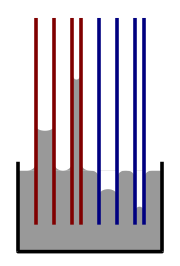Illustration of capillary rise and fall. Red=contact angle less than 90°; blue=contact angle greater than 90°

If a tube is sufficiently narrow and the liquid adhesion to its walls is sufficiently strong, surface tension can draw liquid up the tube in a phenomenon known as capillary action. The height the column is lifted to is given by: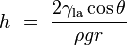$h\ =\ \frac {2\gamma_\mathrm{la} \cos\theta}{\rho g r}$

where

•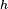$h$ is the height the liquid is lifted,
•$\gamma_\mathrm{la}$ is the liquid-air surface tension,
•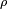$\rho$ is the density of the liquid,
•$r$ is the radius of the capillary,
•$g$ is the acceleration due to gravity,
•$\theta$ is the angle of contact described above. If$\theta$ is greater than 90°, as with mercury in a glass container, the liquid will be depressed rather than lifted.

### Puddles on a surface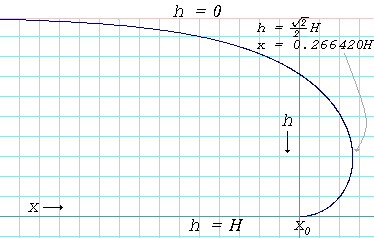Profile curve of the edge of a puddle where the contact angle is 180°. The curve is given by the formula: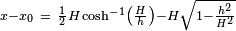$x - x_0 \ = \ \frac {1} {2} H \cosh^{-1}\left(\frac {H}{h}\right) - H \sqrt{1 - \frac{h^2} {H^2}}$ where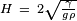$H \ = \ 2 \sqrt{\frac {\gamma} {g \rho}}$

Pouring mercury onto a horizontal flat sheet of glass results in a puddle that has a perceptible thickness. The puddle will spread out only to the point where it is a little under half a centimeter thick, and no thinner. Again this is due to the action of mercury's strong surface tension. The liquid mass flattens out because that brings as much of the mercury to as low a level as possible, but the surface tension, at the same time, is acting to reduce the total surface area. The result is the compromise of a puddle of a nearly fixed thickness.

The same surface tension demonstration can be done with water, lime water or even saline, but only if the liquid does not adhere to the flat surface material. Wax is such a substance. Water poured onto a smooth, flat, horizontal wax surface, say a waxed sheet of glass, will behave similarly to the mercury poured onto glass.

The thickness of a puddle of liquid on a surface whose contact angle is 180° is given by: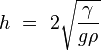$h\ =\ 2 \sqrt{\frac{\gamma} {g\rho}}$

where$h$ is the depth of the puddle in centimeters or meters.$\gamma$ is the surface tension of the liquid in dynes per centimeter or newtons per meter.$g$ is the acceleration due to gravity and is equal to 980 cm/s2 or 9.8 m/s2$\rho$ is the density of the liquid in grams per cubic centimeter or kilograms per cubic meter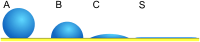Illustration of how lower contact angle leads to reduction of puddle depth

In reality, the thicknesses of the puddles will be slightly less than what is predicted by the above formula because very few surfaces have a contact angle of 180° with any liquid. When the contact angle is less than 180°, the thickness is given by: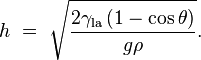$h\ =\ \sqrt{\frac{2\gamma_\mathrm{la}\left( 1 - \cos \theta \right)} {g\rho}}.$

For mercury on glass, γHg = 487 dyn/cm, ρHg = 13.5 g/cm3 and θ = 140°, which gives hHg = 0.36 cm. For water on paraffin at 25 °C, γ = 72 dyn/cm, ρ = 1.0 g/cm3, and θ = 107° which gives hH2O = 0.44 cm.

The formula also predicts that when the contact angle is 0°, the liquid will spread out into a micro-thin layer over the surface. Such a surface is said to be fully wettable by the liquid.

### The breakup of streams into drops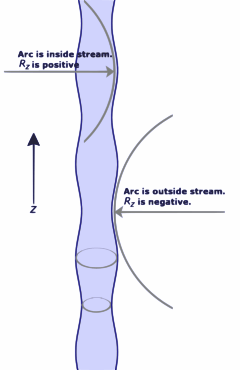Intermediate stage of a jet breaking into drops. Radii of curvature in the axial direction are shown. Equation for the radius of the stream is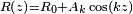$R\left( z \right) = R_0 + A_k \cos \left( kz \right)$, where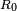$R_0$ is the radius of the unperturbed stream,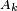$A_k$ is the amplitude of the perturbation,$z$ is distance along the axis of the stream, and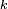$k$ is the wave number

In day-to-day life we all observe that a stream of water emerging from a faucet will break up into droplets, no matter how smoothly the stream is emitted from the faucet. This is due to a phenomenon called the Plateau–Rayleigh instability, which is entirely a consequence of the effects of surface tension.

The explanation of this instability begins with the existence of tiny perturbations in the stream. These are always present, no matter how smooth the stream is. If the perturbations are resolved into sinusoidal components, we find that some components grow with time while others decay with time. Among those that grow with time, some grow at faster rates than others. Whether a component decays or grows, and how fast it grows is entirely a function of its wave number (a measure of how many peaks and troughs per centimeter) and the radii of the original cylindrical stream.

## Data table

Surface tension of various liquids in dyn/cm against air
Mixture %'s are by mass
dyn/cm is equivalent to the SI units of mN/m (milli-Newton per meter)
Liquid Temperature °C Surface tension, γ
Acetic acid 20 27.6
Acetic acid (40.1%) + Water 30 40.68
Acetic acid (10.0%) + Water 30 54.56
Acetone 20 23.7
Diethyl ether 20 17.0
Ethanol 20 22.27
Ethanol (40%) + Water 25 29.63
Ethanol (11.1%) + Water 25 46.03
Glycerol 20 63
n-Hexane 20 18.4
Hydrochloric acid 17.7M aqueous solution 20 65.95
Isopropanol 20 21.7
Liquid Nitrogen -196 8.85
Mercury 15 487
Methanol 20 22.6
n-Octane 20 21.8
Sodium chloride 6.0M aqueous solution 20 82.55
Sucrose (55%) + water 20 76.45
Water 0 75.64
Water 25 71.97
Water 50 67.91
Water 100 58.85

## Images for kidsSurface tension Facts for Kids. Kiddle Encyclopedia.